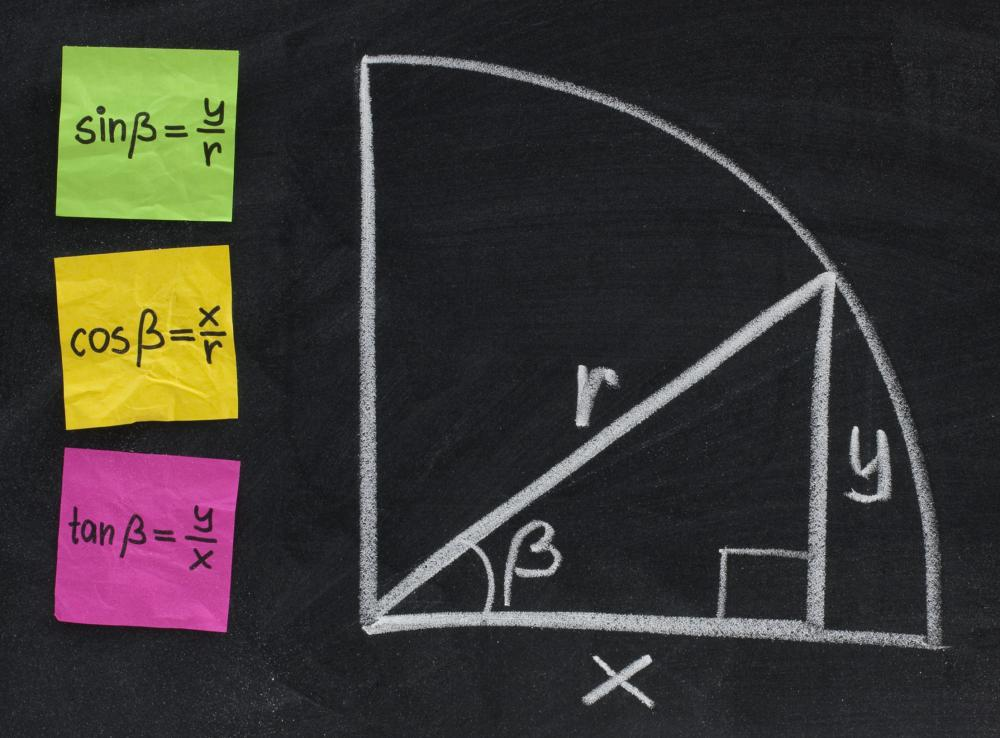# What Is the Angle of Depression?

Christian Petersen
Christian PetersenThe angle of depression can be solved using geometry and trigonometry.

Angle of depression is a term used to describe the angle formed by two lines, one extending from an observer's eye to the horizon and another to an object located some horizontal distance away from and below the observer. The angle of depression is a popular teaching tool in mathematics. A right triangle is formed by connecting three points, with the observer and the object serving as two of the points. The third point is located where the horizontal line from the observer to the horizon intersects with a vertical line extending upwards from the object.

If one or more of the values of the triangle, such as the length of one of the sides or the size of one of the two acute angles is not known, the angle of depression can be calculated using principles of geometry and trigonometry. These exercises are a good way to use practical, everyday situations to illustrate problems that can be difficult for some students to grasp. By creating a framework for the known and unknown values of a problem, students are may be able to visualize the problem more effectively, which helps them to find the correct solution.

Problems involving the angle of depression assume that the line from the observer to the horizon and the ground are parallel. This is useful for situations where the distances are relatively small. When the distances are very large or are part of real world situations, however, rather than hypothetical problems, the curvature of the Earth has an effect, and certain assumptions are no longer valid, especially the one that states that the angle of elevation from the object back to the observer and the angle of depression are equal. The angle of elevation is the angle formed by the ground and a line extending from the object upwards to the observer. As long as the ground and the line extending from the observer to the horizon are parallel, the angles of depression and elevation between the observer and the object are always equal.

The angle of depression is used in surveying, engineering, and geology. Road construction, building projects and civil engineering projects may make use of the angle of depression and the concepts surrounding it to ensure precise construction of many structures as well as proper alignment of things like aqueducts and pipelines. Geologists sometimes use it to describe the arrangement of rock layers relative to the surface of the Earth.

## You might also Like